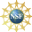# 8. Properties of Curves

## 1. Vector Functions: Position and Plots

In the previous chapter, we used parametric curves to define planar curves in $$\mathbb{R}^2$$ and space curves in $$\mathbb{R}^{3}$$. These are examples of vector functions.

A vector function is a function whose values are vectors.
In $$\mathbb{R}^2$$, a vector function has the form   $$\vec{f}(t)=\langle f_1(t),\,f_2(t)\rangle$$.
In $$\mathbb{R}^{3}$$, a vector function has the form   $$\vec{f}(t)=\langle f_1(t),\,f_2(t),\,f_3(t)\rangle$$.

Throughout this chapter, we will write general formulas for $$3$$ dimensions; the $$2$$ dimensional case, used in many examples, is obtained by dropping one component.

If we think of the resulting vectors as having their tails located at the origin, then the tips trace out a parametric curve. If we regard the parameter as time, then we regard the vector function as the position vector: $\vec{r}(t)=(x(t),y(t),z(t))$ of a particle. A plot of a parametric curve or position vector, is a plot of the points $$\vec{r}(t)$$ for some range of $$t$$'s.

Plot the parametric curve: $\vec{r}(t)=(t\cos t,t\sin t) \qquad \text{for} \qquad 0 \le t \le 4\pi$

If we compare the curve to polar coordinates, $x=r\cos\theta,\quad y=r\sin\theta$ we find $$r=t$$ and $$\theta=t$$. Thus as the angle increases, the radius increases. Thus the plot is the spiral.

There are three examples we will look at repeatedly throughout this chapter: a circle, a helix and a twisted cubic.

Plot the part of the circle of radius $$4$$ centered at the origin in the first $$3$$ quadrants.

Using polar coordinates, the circle may be parametrized as: $\vec{r}(\theta)=(4\cos\theta,4\sin\theta) \qquad \text{for} \qquad 0 \le \theta \le \dfrac{3\pi}{2}$

A circle may also be placed in $$3$$-dimensional space. For example, the circle of radius $$3$$ centered at $$(1,4,2)$$ in the plane $$y=4$$ (which is parallel to the $$xz$$-plane), may be parametrized as: $(x,y,z)=(1+3\cos\theta,4,2+3\sin\theta)$

A helix (half of a DNA molecule) looks like a spring or slinky. If the axis is the along the $$z$$-axis, then the $$x$$ and $$y$$ coordinates may be parametrized just like a circle, while the $$z$$ coordinate increases linearly with the angle. Plot the helix: $\vec{r}(\theta)=(4\cos\theta,4\sin\theta,3\theta) \qquad \text{for} \qquad 0 \le \theta \le 6\pi$ Find the slope of the helix.

Since the angle changes by $$6\pi$$, the helix makes $$3$$ loops. As the curve makes $$1$$ loop, the horizontal displacement is the circumference: $C=2\pi r=2\pi4=8\pi$ while the vertical displacement is: $H=3\theta=3\cdot2\pi=6\pi$ So the slope is: $m=\dfrac{\text{rise}}{\text{run}}=\dfrac{H}{C} =\dfrac{6\pi}{8\pi}=\dfrac{3}{4}$ or the ratio of the coefficient of $$\theta$$ in the $$z$$-component to the radius of the helix.

A twisted cubic is a curve of the form $$\vec{r}(t)=(at,bt^2,ct^{3})$$. Plot the twisted cubic: $\vec{r}(t)=\left(t,t^2,\dfrac{2}{3}t^{3}\right) \qquad \text{for} \qquad -2 \le t \le 2$

PY: Trying new plot format:

Here are $$3$$ plots of the twisted cubic. From the $$z$$-axis, it looks like a quadratic. From the $$y$$-axis, it looks like a cubic. From the $$x$$-axis, it looks like a cusp. (But there is really no sharp point.) You can rotate any of these plots with your mouse.

PY: Old plot format:

Here are $$3$$ plots of the twisted cubic. From the $$z$$-axis, it looks like a quadratic. From the $$y$$-axis, it looks like a cubic. From the $$x$$-axis, it looks like a cusp. (But there is really no sharp point as you can see by rotating it.)View from the $$z$$-axisView from the $$y$$-axis

Match the curves to the plots.

$$a\Longleftrightarrow 4$$
$$b\Longleftrightarrow 3$$
$$c\Longleftrightarrow 1$$
$$d\Longleftrightarrow 2$$

$$a\Longleftrightarrow 4$$
The curve (a) travels around a circle in the $$xz$$-plane while $$y$$ coordinate oscillates $$4$$ times.
$$b\Longleftrightarrow 3$$
For the curve (b), as $$x$$ increases, $$y$$ increases quadratically while $$z$$ increases quartically.
$$c\Longleftrightarrow 1$$
Curve (c) lies in the plane $$z=2$$. If we compare the $$x$$ and $$y$$ cordinates to polar coordinates, $$x=r\cos\theta$$ and $$y=r\sin\theta$$ we find $$r=2+\sin(3t)$$ and $$\theta=t$$ which is the polar equation of a $$3$$ leaf clover.
$$d\Longleftrightarrow 2$$
Curve (d) is a spiral in the $$xy$$ coordinates while $$z$$ increases.

$$a)\qquad \vec{r}(t)=(4\cos t,\sin(4t),4\sin t)$$
$$b)\qquad \vec{r}(t)=(t,t^2,t^4)$$
$$c)\qquad \vec{r}(t)=\left((2+\sin(3t))\cos t,(2+\sin(3t))\sin t,2\right)$$
$$d)\qquad \vec{r}(t)=(t\cos t,t\sin t,t^2)$$

PY: New plot format:
PY: Old plot format: